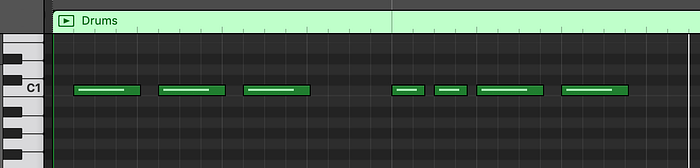# How to Generate Techno Music using Deep Learning

Originally Published on Medium.com on July 26th, 2019This visual demonstrates a simple pattern stored in a MIDI file for a drum, using GarageBand. A song will usually be a combination of multiple MIDI structures, each representing a different instrument.

## Data Preparation

```notes = []for file in glob.glob("midi_songs/*.mid"):
midi = converter.parse(file)print("Parsing %s" % file)notes_to_parse = None    try:
s2 = instrument.partitionByInstrument(midi)
notes_to_parse = s2.parts.recurse()
except:
notes_to_parse = midi.flat.notes    for element in notes_to_parse:
if isinstance(element, note.Note):
notes.append(str(element.pitch))
elif isinstance(element, chord.Chord):
notes.append('.'.join(str(n) for n in element.normalOrder))with open('data/notes', 'wb') as filepath:
pickle.dump(notes, filepath)```
`note_to_int = dict((note, number) for number, note in enumerate(pitchnames))`
```model = Sequential()
512,
input_shape=(network_input.shape, network_input.shape),
return_sequences=True
))
model.compile(loss=’categorical_crossentropy’, optimizer=’rmsprop’)```

## Training the Model

`model.fit(network_input, network_output, epochs=30, batch_size=64, callbacks=callbacks_list)`
```filepath = “weights-improvement-{epoch:02d}-{loss:.4f}-bigger.hdf5”
checkpoint = ModelCheckpoint(
filepath,
monitor=’loss’,
verbose=0,
save_best_only=True,
mode=’min’
)
callbacks_list = [checkpoint]```

## Music Generation

```with open('data/notes', 'rb') as filepath:
```sequence_length = 100
network_input = []
output = []
for i in range(0, len(notes) - sequence_length, 1):
sequence_in = notes[i:i + sequence_length]
sequence_out = notes[i + sequence_length]
network_input.append([note_to_int[char] for char in sequence_in])
output.append(note_to_int[sequence_out])```This diagram demonstrates how the model generates music notes. In this example, the input sequence is ABCDE. The model predicts that the next note is F so in the next iteration we remove A and append F. The process is then repeated.
```prediction_output = []    for note_index in range(500):
prediction_input = numpy.reshape(pattern, (1, len(pattern), 1))
prediction_input = prediction_input / float(n_vocab)prediction = model.predict(prediction_input, verbose=0)index = numpy.argmax(prediction)
result = int_to_note[index]
prediction_output.append(result) pattern.append(index)
pattern = pattern[1:len(pattern)]```
```for pattern in prediction_output:
if ('.' in pattern) or pattern.isdigit():
notes_in_chord = pattern.split('.')
notes = []
for current_note in notes_in_chord:
new_note = note.Note(int(current_note))
new_note.storedInstrument = instrument.SnareDrum()
notes.append(new_note)
new_chord = chord.Chord(notes)
new_chord.offset = offset
output_notes.append(new_chord)
else:
new_note = note.Note(pattern)
new_note.offset = offset
new_note.storedInstrument = instrument.SnareDrum()
output_notes.append(new_note)offset += 0.5```

This site uses Akismet to reduce spam. Learn how your comment data is processed.